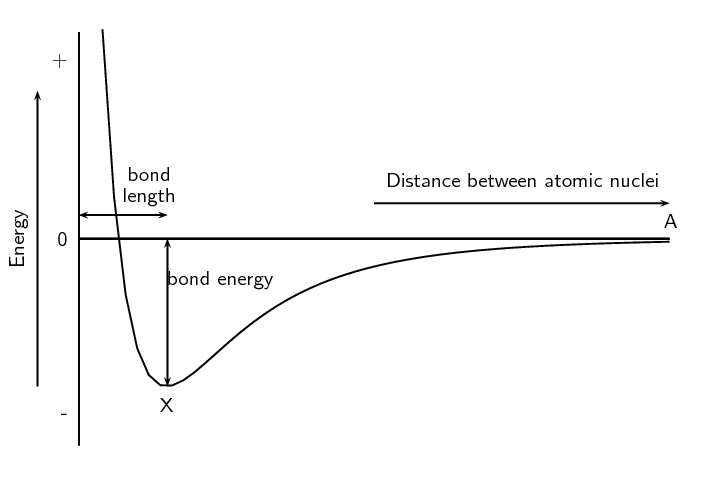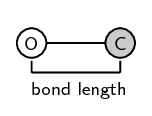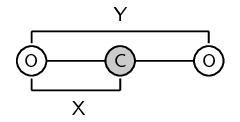Home Practice
For learners and parents For teachers and schools
Textbooks
Full catalogue
Pricing SupportLog in

We think you are located in United States. Is this correct?

# 3.4 Energy and bonding

## 3.4 Energy and bonding (ESBMJ)

As we saw earlier in the chapter we can show the energy changes that occur as atoms come together (Figure 3.5). Shown below is the same image but this time with two extra pieces of information: the bond energy and the bond length.Figure 3.9: Graph showing the change in energy that takes place as atoms move closer together.
Bond length
The distance between the nuclei of two adjacent atoms when they bond.
Bond energy
The amount of energy that must be added to the system to break the bond that has formed.

It is important to remember that bond length is measured between two atoms that are bonded to each other. The following diagrams show the bond length for $$\text{CO}$$ and for $$\text{CO}_{2}$$. The grey circle represents carbon and the white circle represents oxygen.Figure 3.10: The bond length for carbon monoxide ($$\text{CO}$$).Figure 3.11: The bond length for each $$\text{C}-\text{O}$$ bond in carbon dioxide ($$\text{CO}_{2}$$) is indicated by X. Y is not the bond length.

A third property of bonds is the bond strength. Bond strength means how strongly one atom attracts and is held to another. The strength of a bond is related to the bond length, the size of the bonded atoms and the number of bonds between the atoms. In general:

• the shorter the bond length, the stronger the bond between the atoms.

• the smaller the atoms involved, the stronger the bond.

• the more bonds that exist between the same atoms, the stronger the bond.

temp text